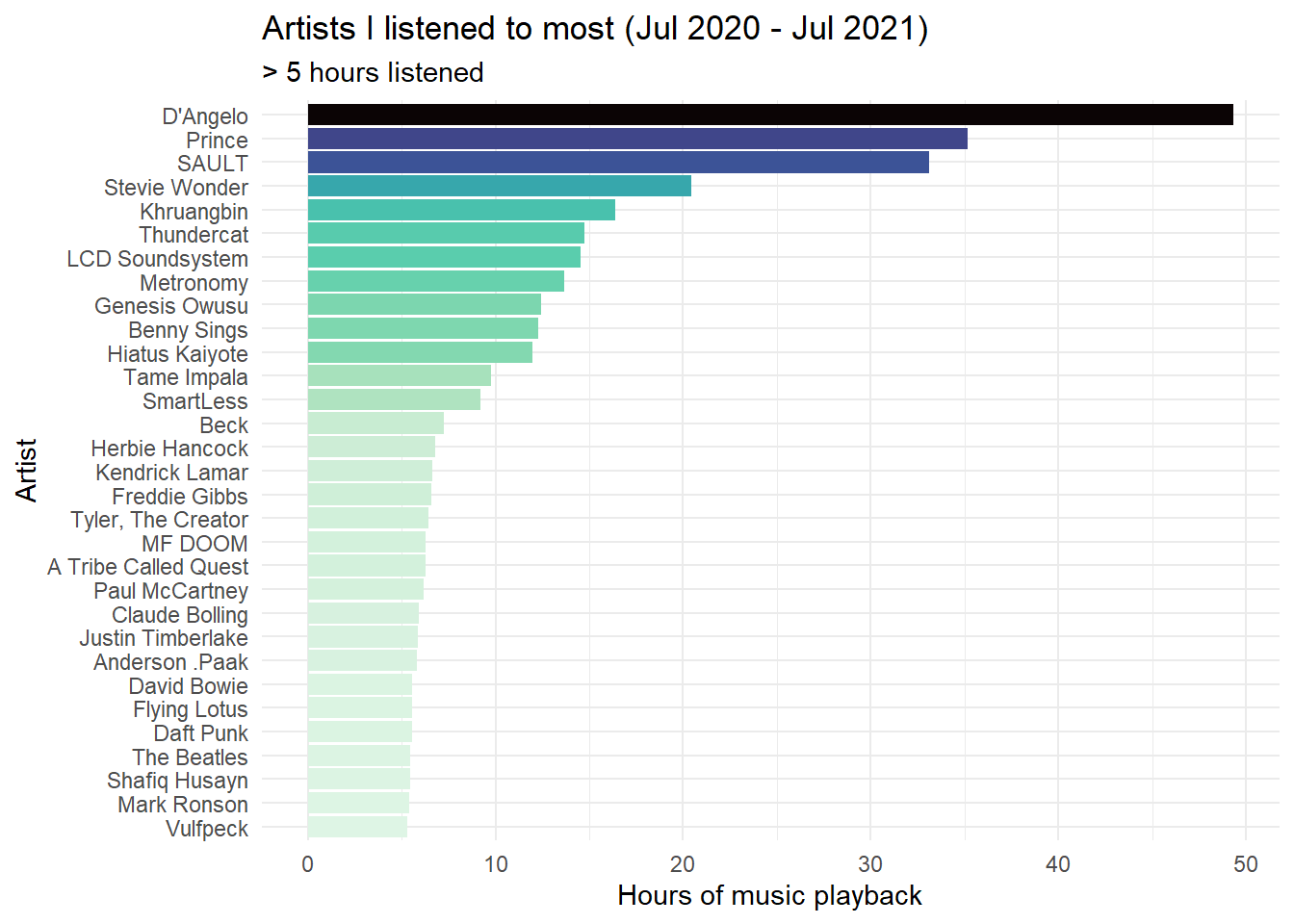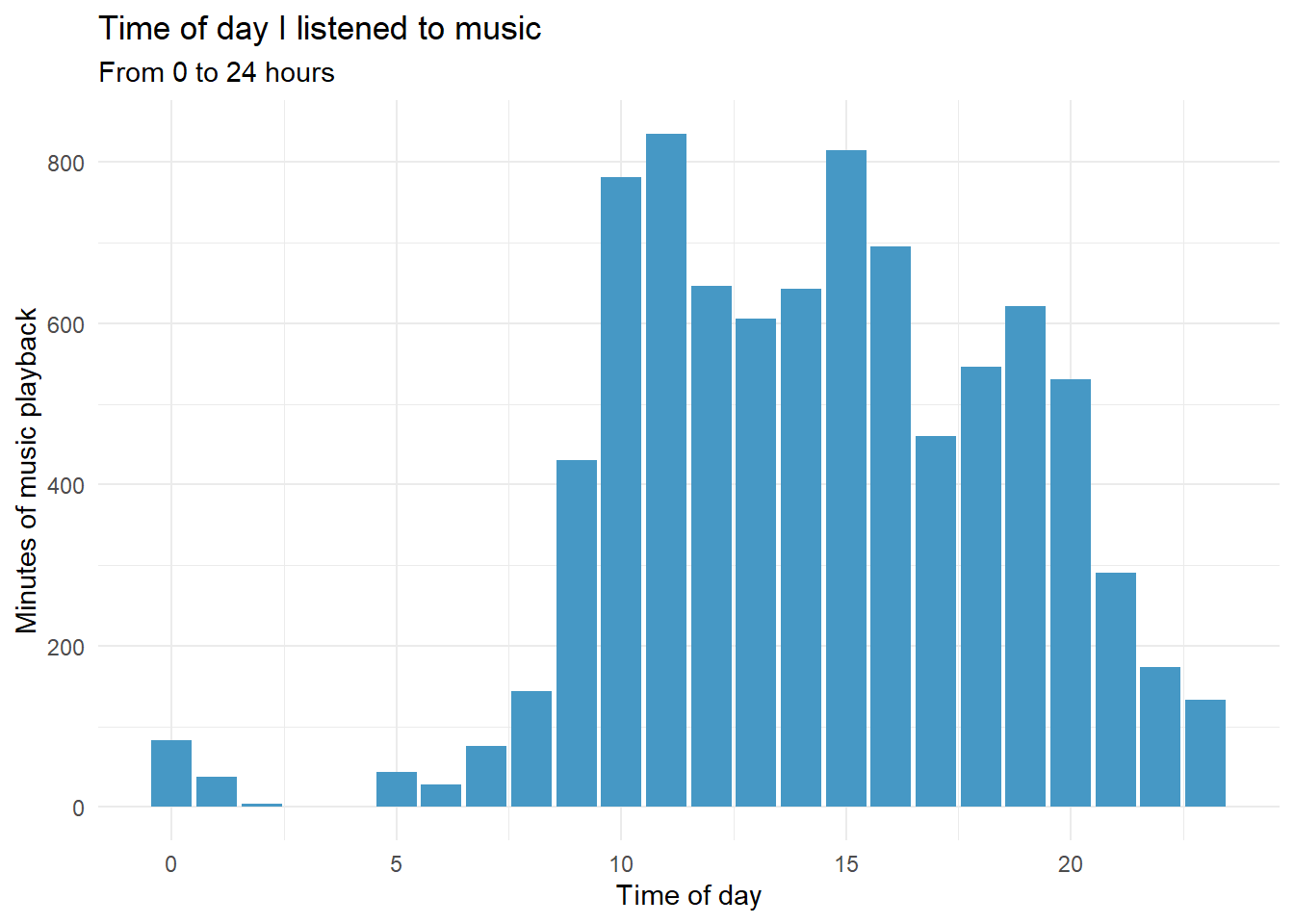# My Listening

What have I been listening to? Let’s find out…using plots!

music
dataviz
Published

August 30, 2021

Spotify let’s you download your listening history without much fuss. Thanks to some excellent posts like this one and some really cool packages like spotifyr, it’s also easy to learn how to download your Spotify data and make some nice visualisations in R.

So, I thought I would try it out!

``````# packages
library(jsonlite)
library(lubridate)
library(gghighlight)
library(spotifyr)
library(tidyverse)
library(kableExtra)
library(plotly)
library(viridis)
library(here)``````
``````# Importing data

# Import the stream data from Spotify which are in JSON-format.
# Create a month variable by flooring the stream date column.
df <- bind_rows(
as_tibble(fromJSON("private_data/MyData/StreamingHistory0.json")),
as_tibble(fromJSON("private_data/MyData/StreamingHistory1.json")),
as_tibble(fromJSON("private_data/MyData/StreamingHistory2.json"))
) %>%
mutate_at("endTime", ymd_hm) %>%
mutate(endTime = endTime + hours(10)) %>%
mutate(date = floor_date(endTime, "day") %>%
as_date, seconds = msPlayed / 1000, minutes = seconds / 60)``````

# Hours of listening per week

``````# Playback activity per week & hours

streamingHours <- df %>%
group_by(date = floor_date(date, "week")) %>%
summarise(hours = sum(minutes) / 60) %>%
arrange(date) %>%

ggplot(aes(x = date, y = hours)) +
geom_col(aes(fill = hours), alpha = 0.9) +
scale_fill_viridis(option = "D", direction = -1) +
labs(x= "Date", y= "Hours of music playback") +
ggtitle("My weekly listening on Spotify (Jul 2020 - Jul 2021)") +
theme_minimal()

ggplotly(streamingHours)``````

# Artists I listen to most

``````minutesMostListened <- df %>%
group_by(artistName) %>%
summarise(hoursListened = ((sum(minutes)) / 60) %>% round(., 2)) %>%
filter(hoursListened >= 5) %>%

ggplot(aes(x = reorder(artistName, hoursListened), y = hoursListened)) +
geom_col(aes(fill = hoursListened)) +
scale_fill_viridis(option = "G", direction = -1) +
labs(x= "Artist", y= "Hours of music playback") +
ggtitle("Artists I listened to most (Jul 2020 - Jul 2021)",
subtitle = "> 5 hours listened") +
theme_minimal() +
theme(legend.position = "none") +
coord_flip()

minutesMostListened``````Note: This section requires that you get a Client ID and secret number from Spotify. See this section from Saúl Buentello’s blog post to learn how.

``````# To do this yourself you need to get the following:
library(spotifyr)
Sys.setenv(SPOTIFY_CLIENT_ID = Sys.getenv("CLIENTID"))
Sys.setenv(SPOTIFY_CLIENT_SECRET = Sys.getenv("CLIENTSECRET"))

access_token <- get_spotify_access_token()``````

# When do I listen to music?

``````# When am I listening to music?
hoursDay <- df %>%
mutate(
hour = hour(endTime),
weekday = wday(date, label = TRUE)
) %>%
group_by(hour, weekday) %>%
summarise(minutesListened = sum(minutes))

hoursDay %>%
ggplot(aes(x = hour, y = minutesListened)) +
stat_summary(fun = "mean", geom = "bar", fill = "#4698c5") +
labs(x = "Time of day", y = "Minutes of music playback") +
ggtitle("Time of day I listened to music",
"From 0 to 24 hours") +
theme_minimal()``````